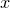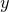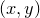# 8.14 Three-dimensional plotting

Three-dimensional graphs may be produced by placing the modifier 3d immediately after the plot command, as demonstrated by the following simple example which draws a helix:

set key below
set size 8 ratio 0.6 zratio 0.6
set grid
plot 3d sin(x):cos(x) with lw 3 col hsb(x/20+0.5,0.9,0.8)Many plot styles take additional columns of data when used on three-dimen­sional plots, reading in three values for the,andcoordinates of each datapoint, where previously onlyandcoordinates were required. In the above example, the lines plot style is used, which takes three columns of input data when used on three-dimensional plots, as compared to two on two-dimensional plots. The descriptions of each plot style in Section 8.2 includes information on the number of columns of data required for two- and three-dimensional plots.

The example above also demonstrates that the set size command takes an additional aspect ratio zratio which affects three-dimensional plots; whereas the aspect ratio ratio determines the ratio of the lengths of the-axes of plots to their-axes, the aspect ratio zratio determines the ratio of the lengths of the-axes of plots to their-axes.

The angle from which three-dimensional plots are viewed can be set using the set view command. This should be followed by two angles, which can either be expressed in degrees, as dimensionless numbers, or as quantities with physical units of angle:

```set view 60,30

set unit angle nodimensionless
The orientationcorresponds to having the-axis horizontal, the-axis vertical, and the-axis directed into the page. The first angle supplied to the set view command rotates the plot in theplane, and the second angle tips the plot up in the plane containing the-axis and the normal to the user’s two-dimensional display.